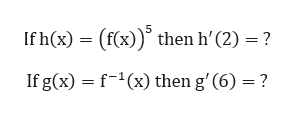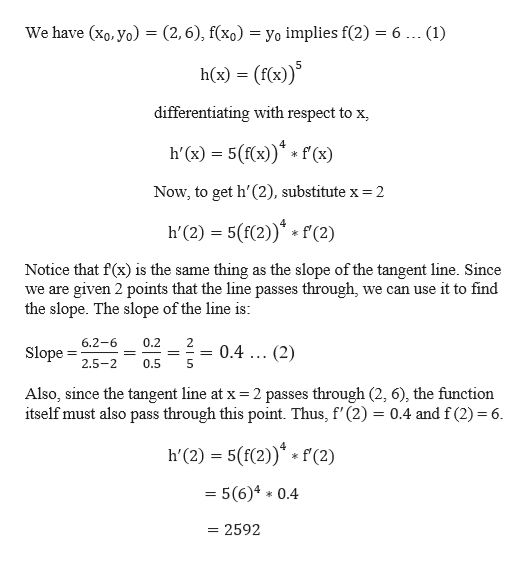Question
21 views

Let (x0,y0)=(2,6) and (x1,y1)= (2.5,6.2). Use the following graph of the function f to find the indicated derivatives.

If h(x)=(f(x))^5 then

h'(2)=?

If g(x)=f(^-1) (x) then

g'(6)=?

check_circle

Step 1

Given points that lie on tangent are:

Step 2

To find:help_outlineImage TranscriptioncloseIf h(x) (fx)) then h' (2) =? If g(x) f1(x) then g'(6) = ? fullscreen
Step 3

a) To begin ...help_outlineImage TranscriptioncloseWe have (xo, yo) = (2,6), f(xo) = yo implies f(2) = 6 ... (1) h(x) (f(x)) differentiating with respect to x h'(x) 5(x)(x) = Now, to get h'(2), substitute x = 2 h'(2) 5(f(2))(2) Notice that f(x) is the same thing as the slope of the tangent line. Since we are given 2 points that the line passes through, we can use it to find the slope. The slope of the line is: 0.2 6.2-6 2 ===0.4 ... (2) 0.5 Slope 2.5-2 Also, since the tangent line at x 2 passes through (2, 6), the function itself must also pass through this point. Thus, f' (2) 0.4 and f (2)=6 h'(2) 5((2))f (2) = 5(6)4 * 0.4 = 2592 fullscreen

### Want to see the full answer?

See Solution

#### Want to see this answer and more?

Solutions are written by subject experts who are available 24/7. Questions are typically answered within 1 hour.*

See Solution
*Response times may vary by subject and question.
Tagged in

### Functions